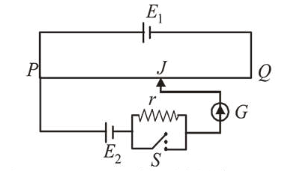# A potentiometer wire P Q of 1 m length is connected to a standard

Question:

A potentiometer wire $P Q$ of $1 \mathrm{~m}$ length is connected to a standard cell $E_{1}$. Another cell $E_{2}$ of emf $1.02 \mathrm{~V}$ is connected with a resistance ' $r$ ' and switch $S$ (as shown in figure). With switch $S$ open, the null position is obtained at a distance of $49 \mathrm{~cm}$ from $Q$. The potential gradient in the potentiometer wire is:1. (1) $0.02 \mathrm{~V} / \mathrm{cm}$

2. (2) $0.01 \mathrm{~V} / \mathrm{cm}$

3. (3) $0.03 \mathrm{~V} / \mathrm{cm}$

4. (4) $0.04 \mathrm{~V} / \mathrm{cm}$

Correct Option: 1

Solution:

(1) Potential gradient, $x=\frac{\text { Potential drop }}{\text { length }}$

Here, Potential drop $=1.02$

Balancing length from $\mathrm{P}=100-49$

$\therefore x=\frac{1.02}{100-49}=0.02 \mathrm{volt} / \mathrm{cm}$